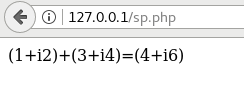Announcing BigAnimal: Fully managed PostgreSQL in the Cloud
Postgres Build 2021: 30 Nov to 1 Dec# How to call PostgreSQL stored procedures from a PHP application

Abbas Butt2/19/2020
Developers

SUMMARY: This tutorial provides instructions and an example for calling a PostgreSQL stored procedure from a PHP application.

1. Creating the PostgreSQL stored procedure

2. Creating the example program in PHP

3. Running the example program

### Prerequisites

For this demonstration we will be using the following software versions:

1. CentOS Linux release 7.5.1804
2. PostgreSQL 12
3. Apache 2.4
4. PHP 7

## 1. Creating the PostgreSQL stored procedure

The example program will call the following stored procedure, which adds two complex numbers and returns the result in INOUT parameters. Let's start by creating a stored procedure:

``````CREATE OR REPLACE PROCEDURE add_complex(IN real_1 INTEGER,

IN imaginary_1 INTEGER,

IN real_2 INTEGER,

IN imaginary_2 INTEGER,

INOUT real_res INTEGER,

INOUT imaginary_res INTEGER)

AS \$\$

BEGIN

real_res := real_1 + real_2;

imaginary_res := imaginary_1 + imaginary_2;

END;

\$\$

LANGUAGE plpgsql;``````

## 2. Creating the example program in PHP

Now, let’s call this stored procedure using PHP.

``````[abbas@localhost html]\$ pwd

/var/www/html``````

Create the following file sp.php at the above location:

### sp.php

``````<?php

\$dbconn = pg_connect("host=127.0.0.1 user=postgres dbname=postgres port=5432")

or die('Could not connect: ' . pg_last_error());

\$real_1 = 1;

\$imaginary_1 = 2;

\$real_2 = 3;

\$imaginary_2 = 4;

\$result = pg_query_params(\$dbconn, 'CALL add_complex(\$1, \$2, \$3, \$4, NULL, NULL)',

array(\$real_1, \$imaginary_1, \$real_2, \$imaginary_2))

or die('Unable to CALL stored procedure: ' . pg_last_error());

\$row = pg_fetch_row(\$result);

\$res_real = \$row;

\$res_imaginary = \$row;

pg_free_result(\$result);

echo "<p>(\$real_1+i\$imaginary_1)+(\$real_2+i\$imaginary_2)=(\$res_real+i\$res_imaginary)</p>";

pg_close(\$dbconn);

?>

[abbas@localhost html]\$ pwd

/var/www/html``````

Update the file index.html at the above location with the content as shown below:

### index.html

``````<html>

<title>Stored Procedure from a PHP application</title>

<body>

<p><a href="sp.php">Execute SP to add two complex numbers</a></p>

</body>

</html>``````

## 3. Running the example program

Now open the file in browser 127.0.0.1 and click on “Execute SP to add two complex numbers”: